# Punjab Board (PSEB) Question Paper for Class 10th Maths 2017 In PDF

## PSEB Matriculation (10th Standard) Maths Exam Question Papers 2017 with Solutions – Free Download

PSEB (Punjab board) Annual Exam Class 10 Maths 2017 Question Paper (A) with solutions are available at BYJU’S. Students can get the previous years question papers of Punjab board annual exam at BYJU’S to practice as a part of their preparation. The solutions for these previous years of pseb class 10 maths question papers are available in a free downloadable pdf format. Practising more question papers of PSEB Class 10 Maths will help the students in scoring maximum marks in their final exam. Scoring more marks in Mathematics of Class 10 requires more practice of all the important Maths concepts and questions. Thus, Punjab Board (PSEB) Question Paper for Class 10th Maths 2017 In PDF with Solutions is the best resource to prepare most efficiently for the board exams.

### PSEB Class 10 Mathematics Question Paper 2017 Paper A with Solutions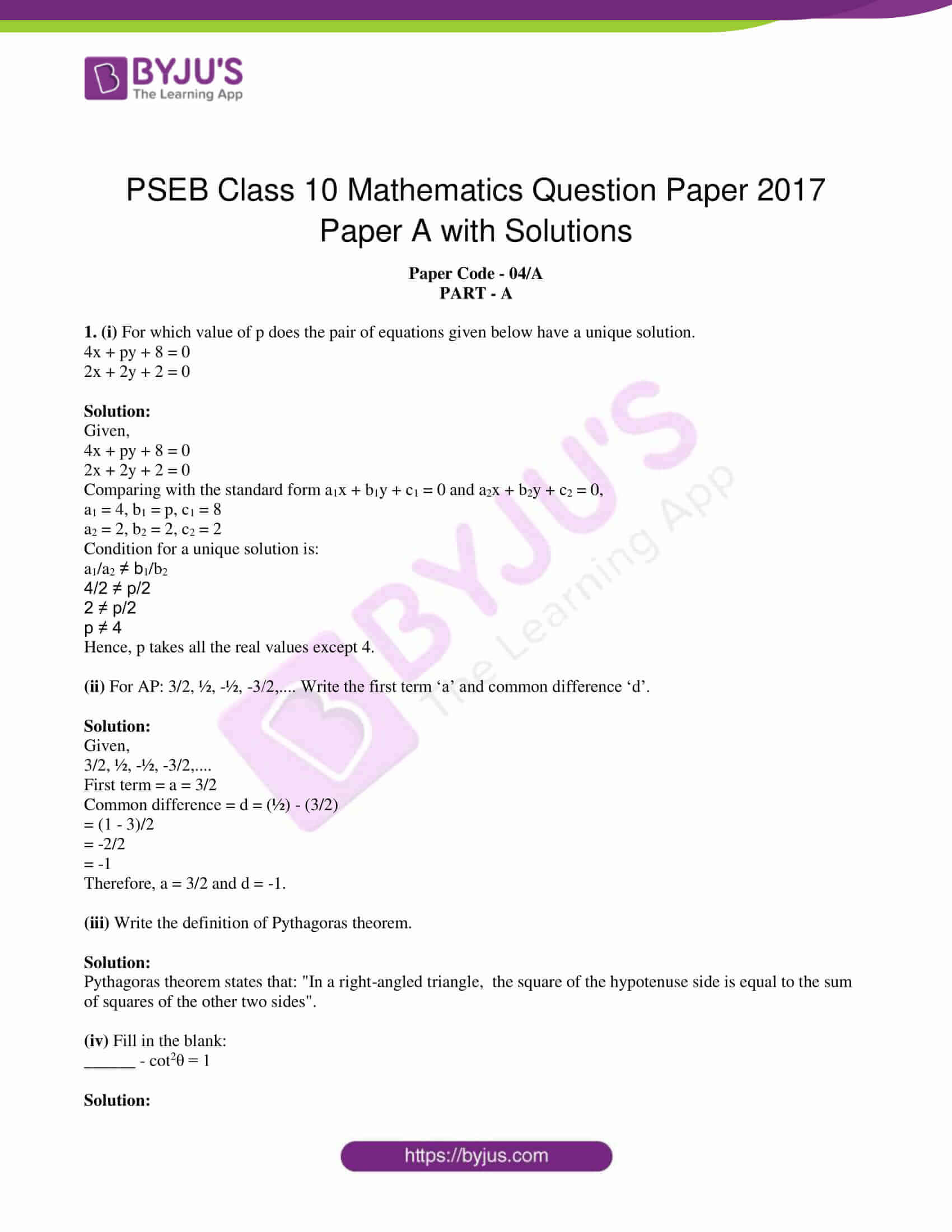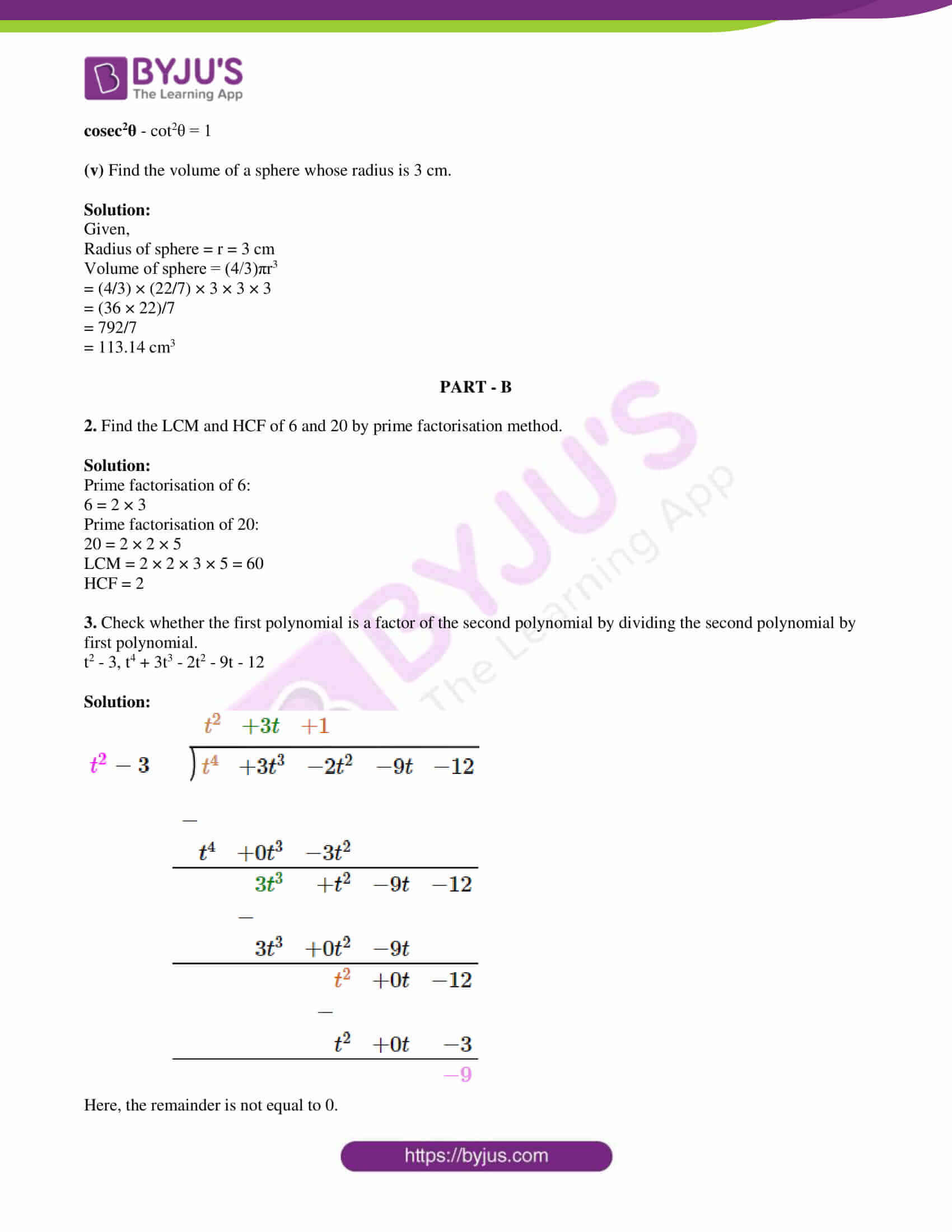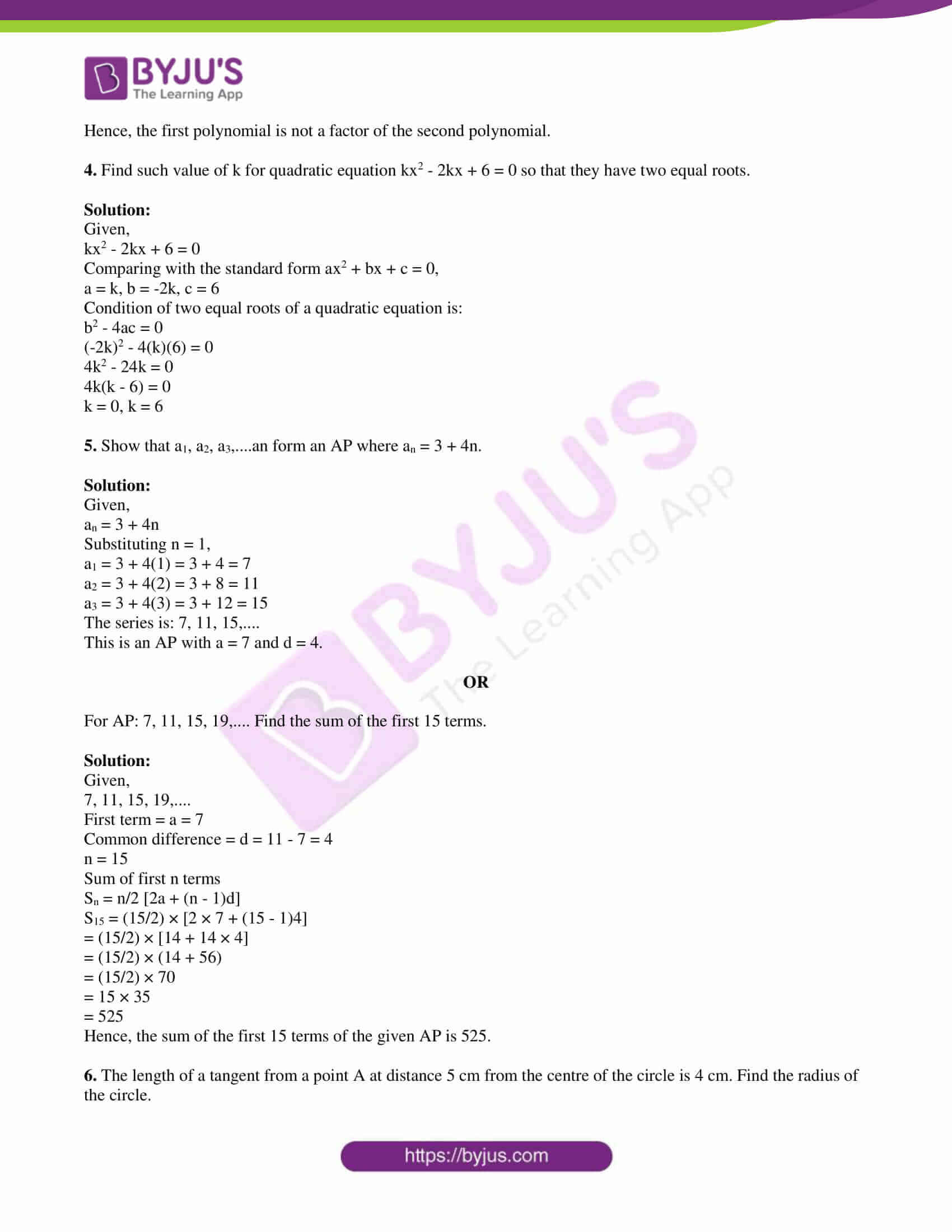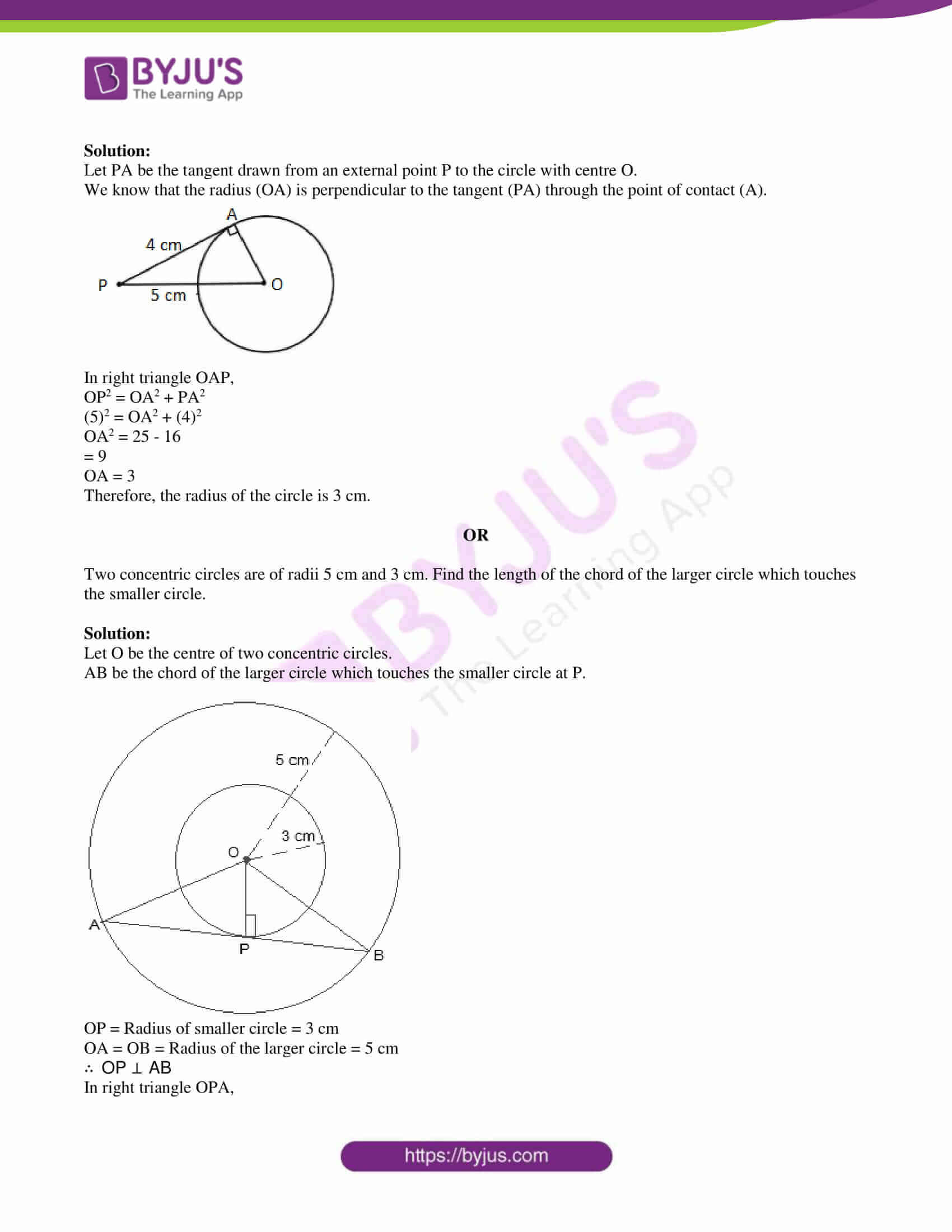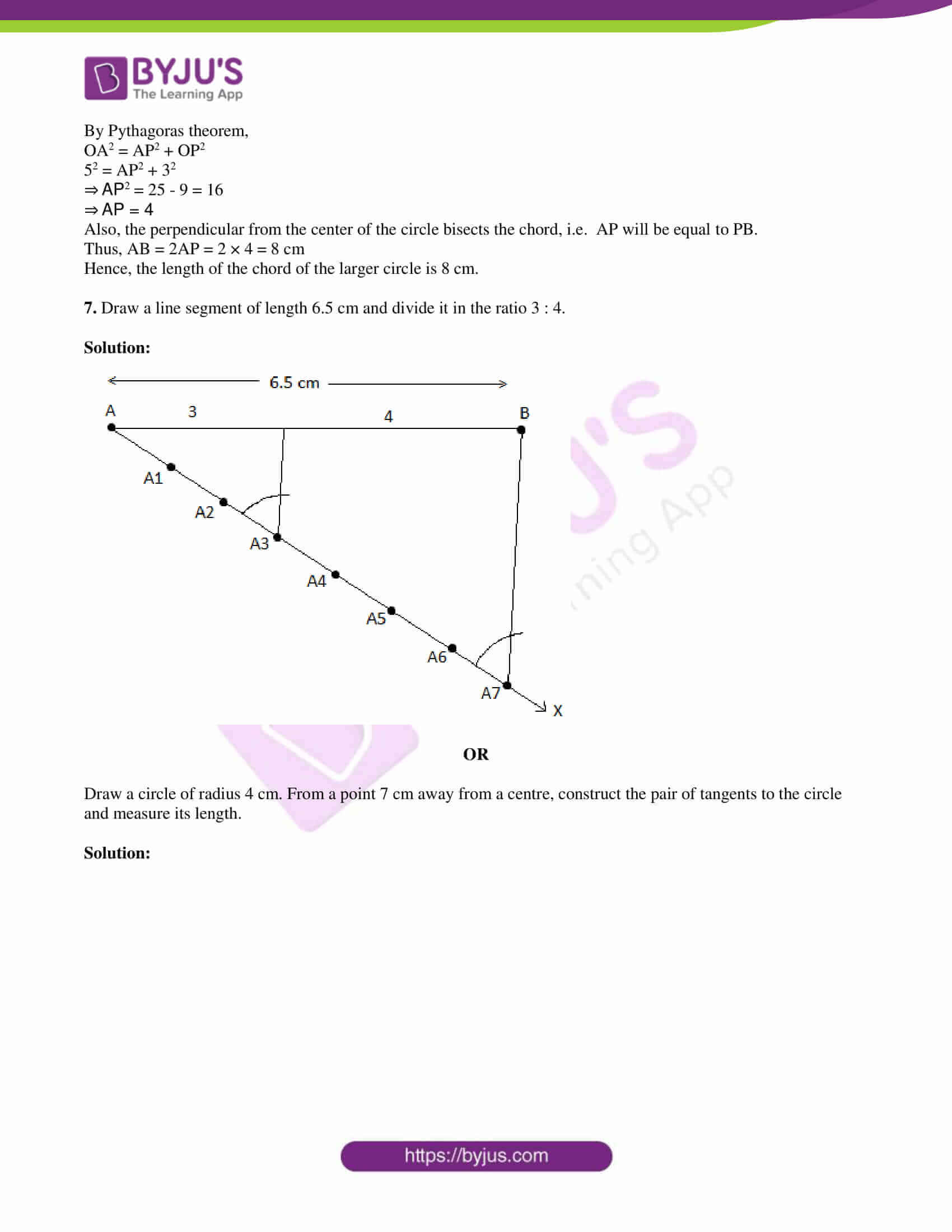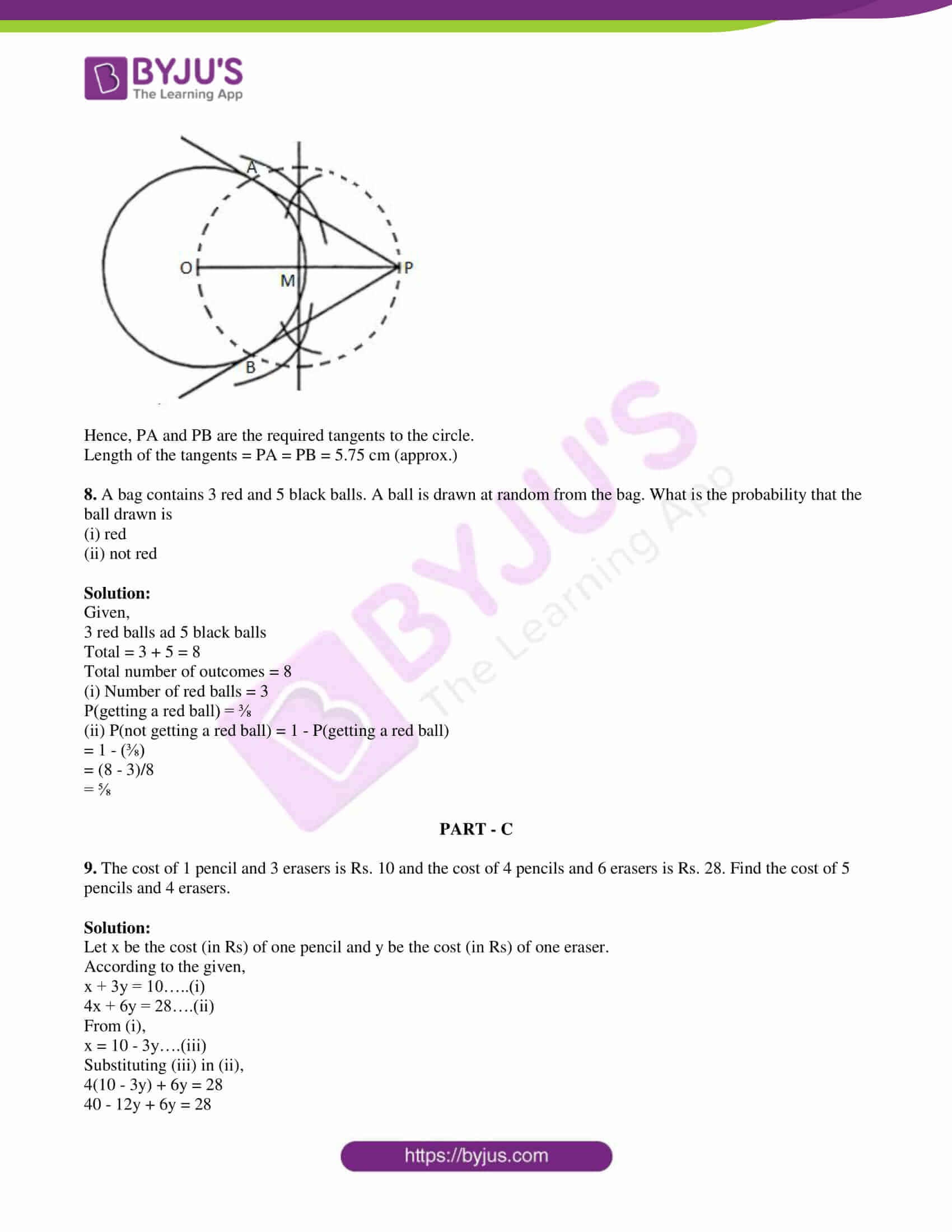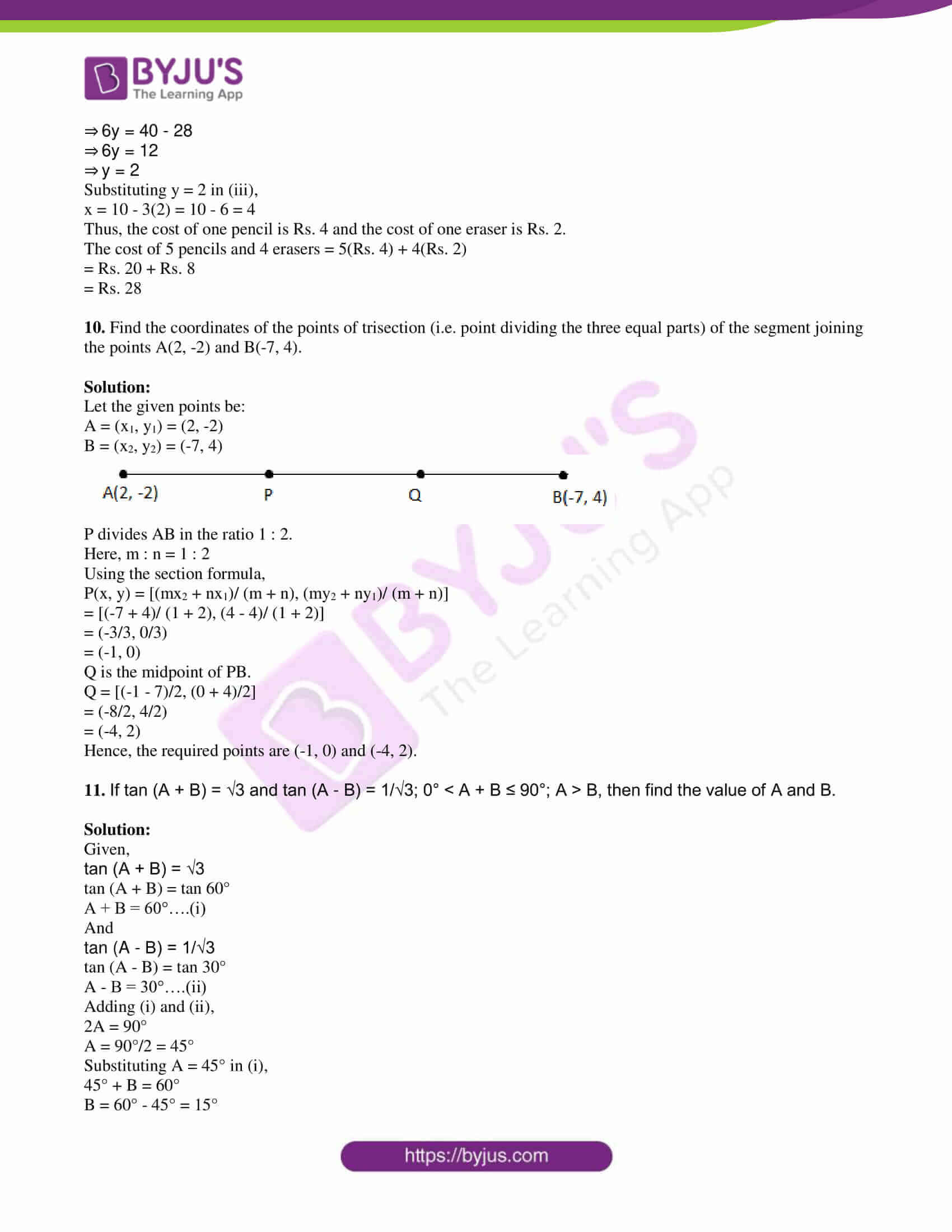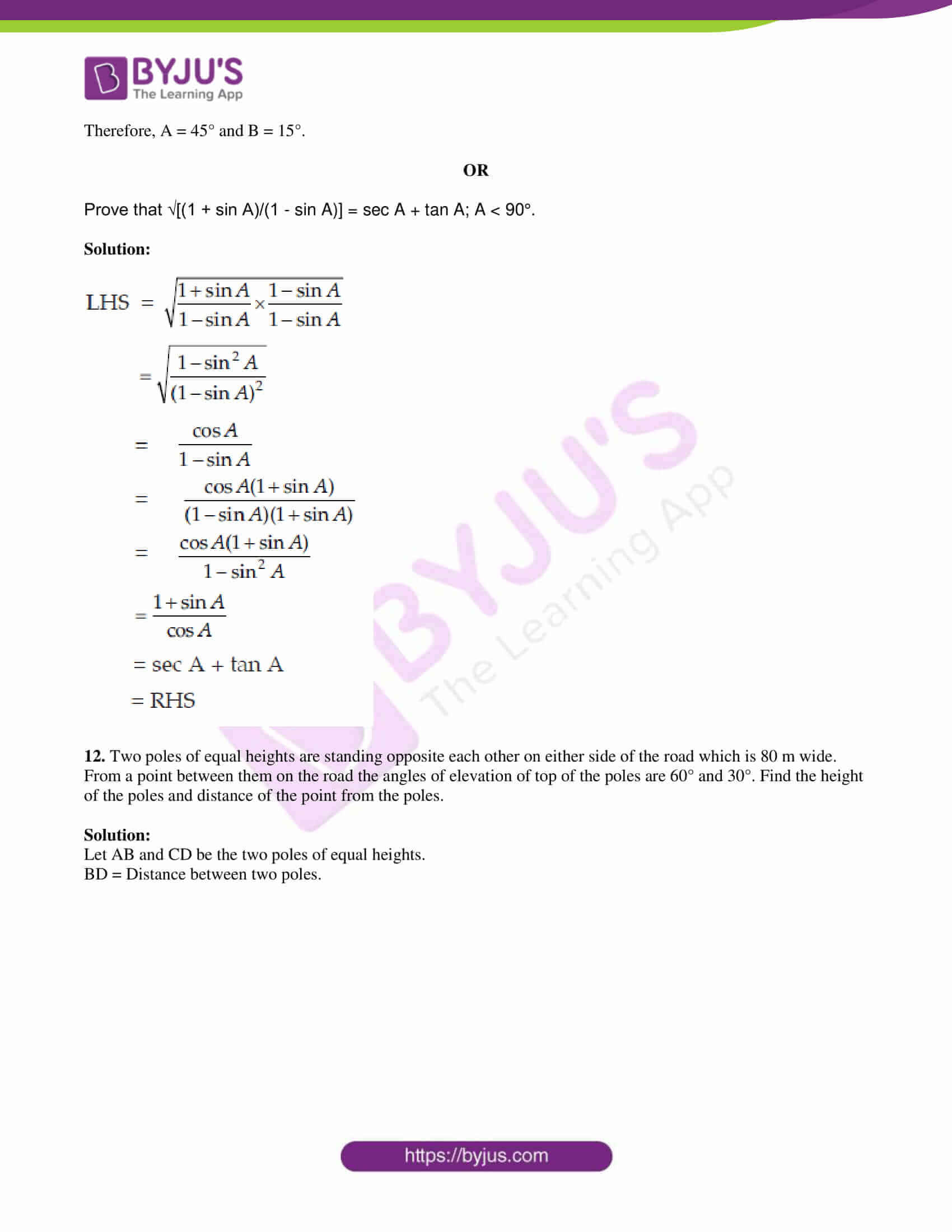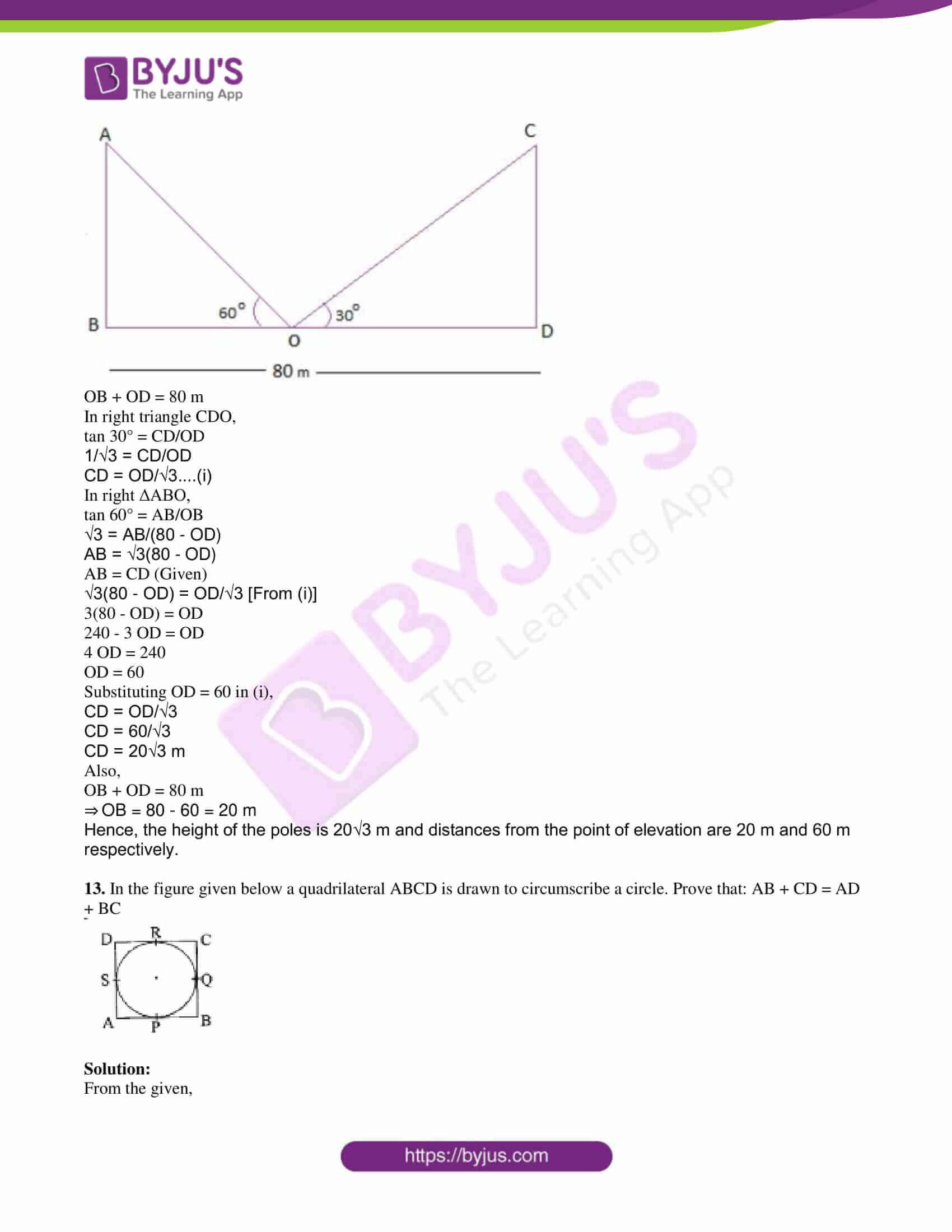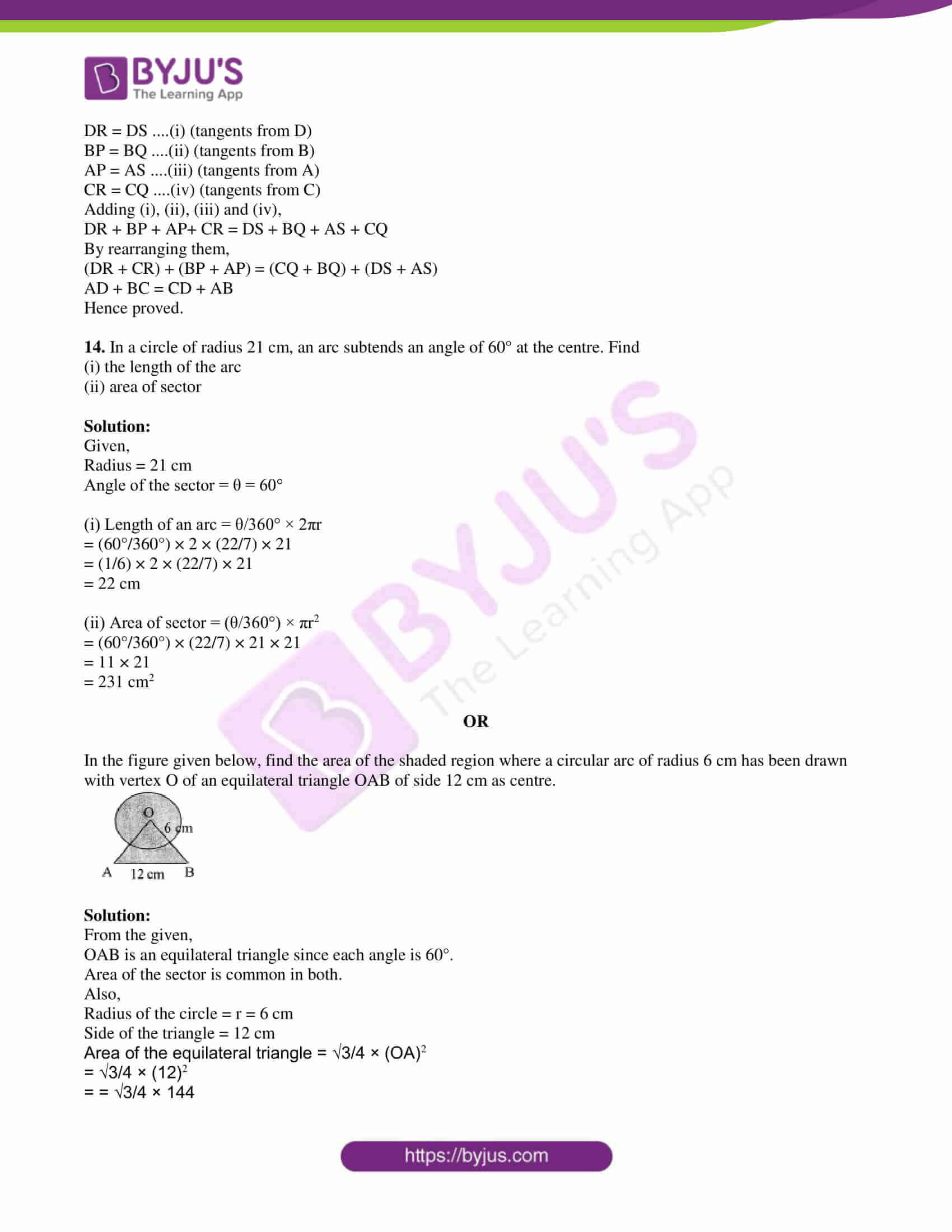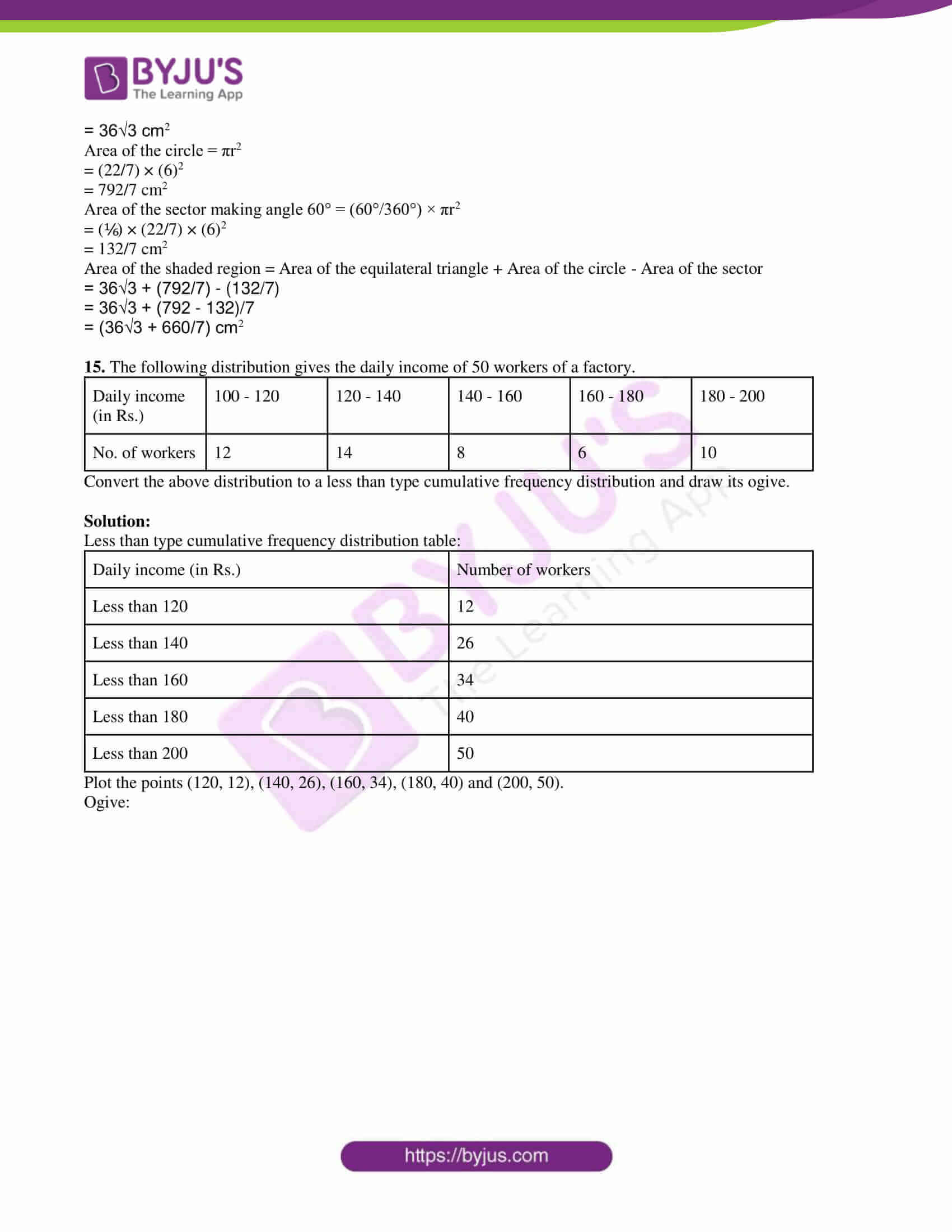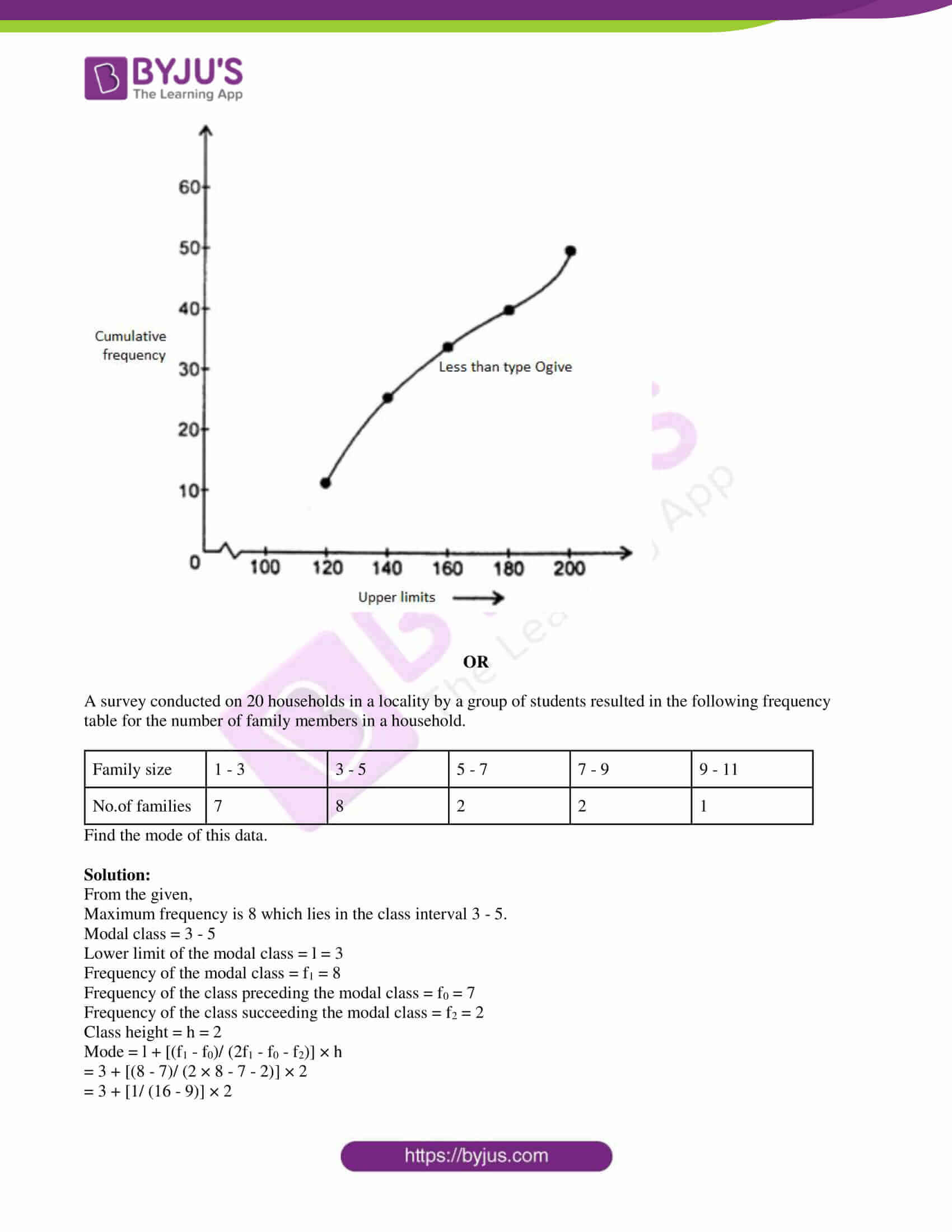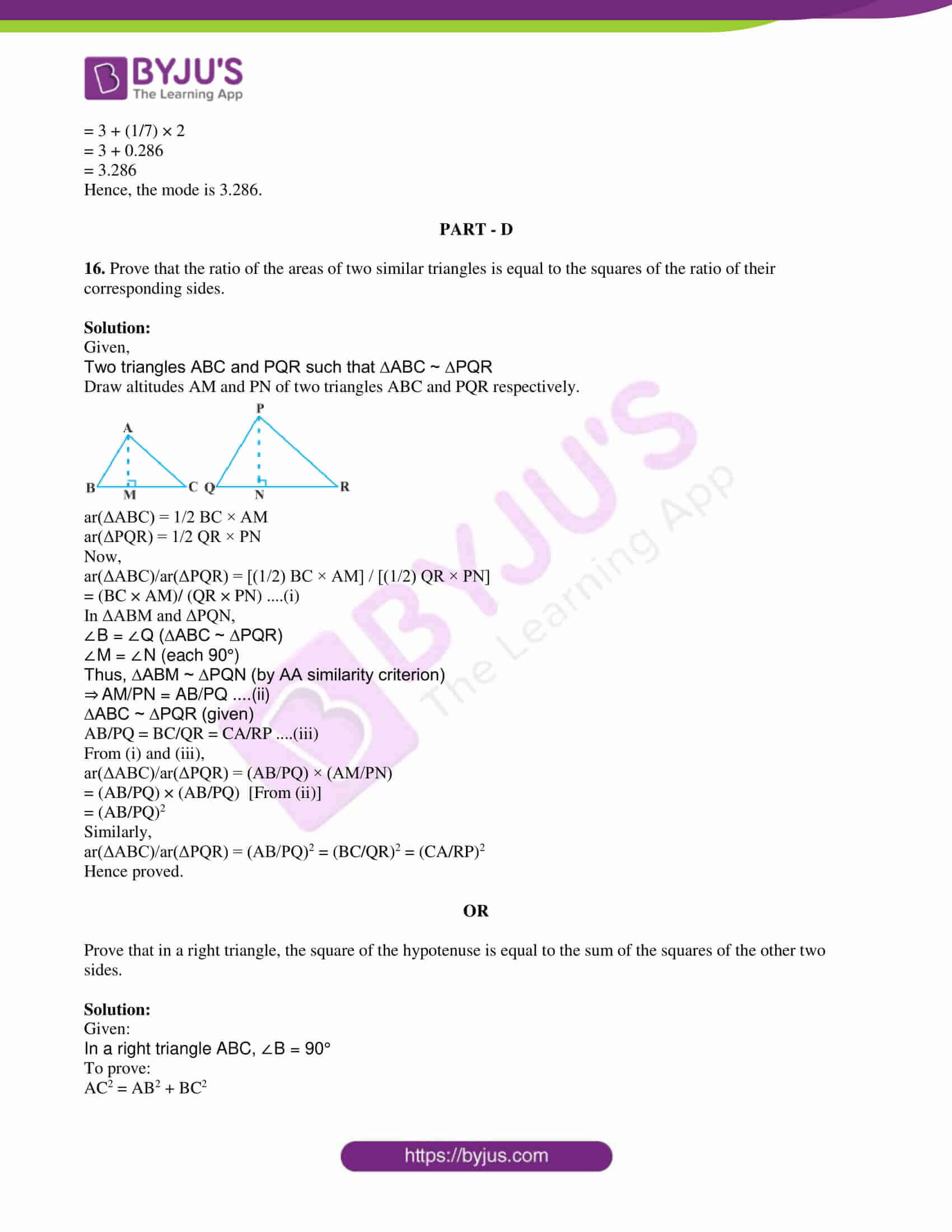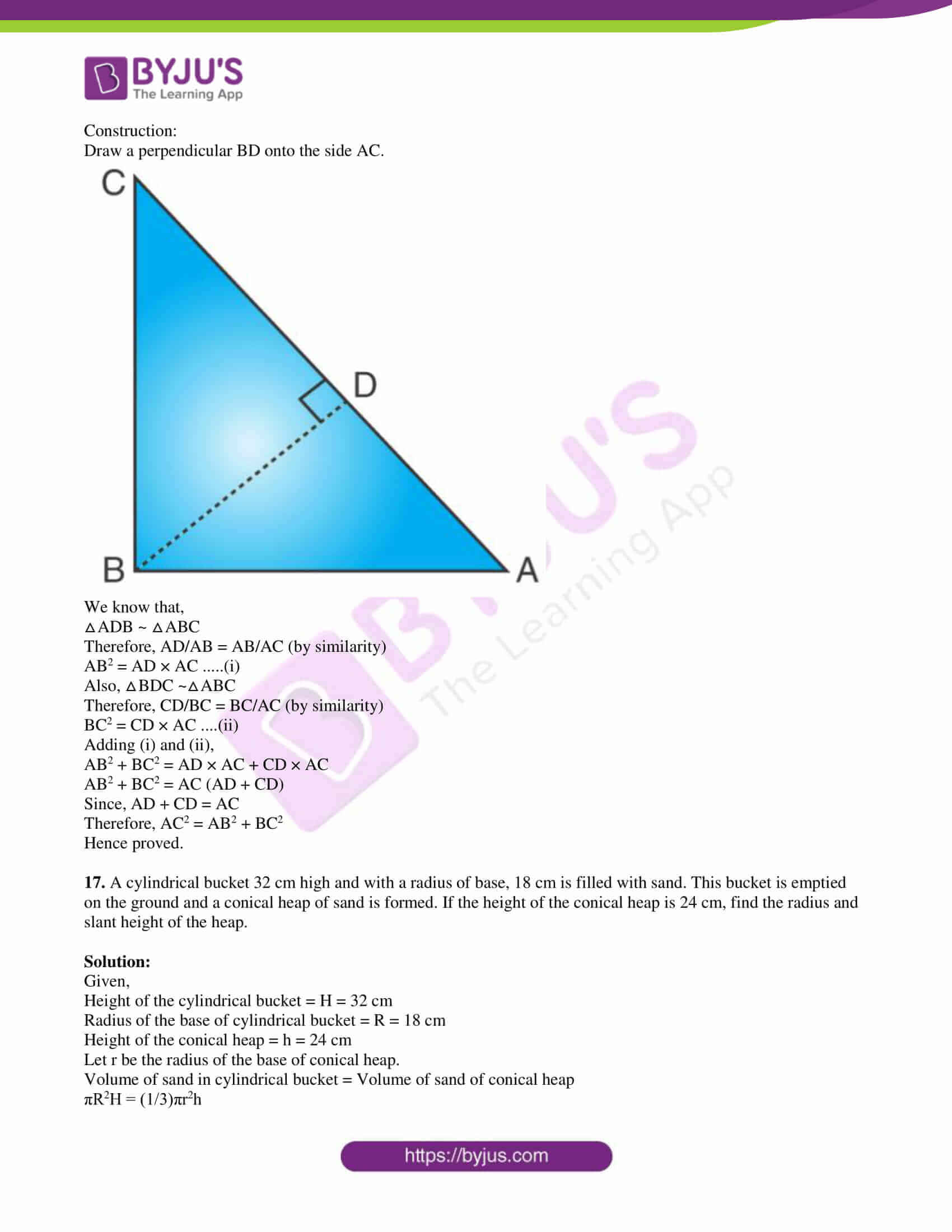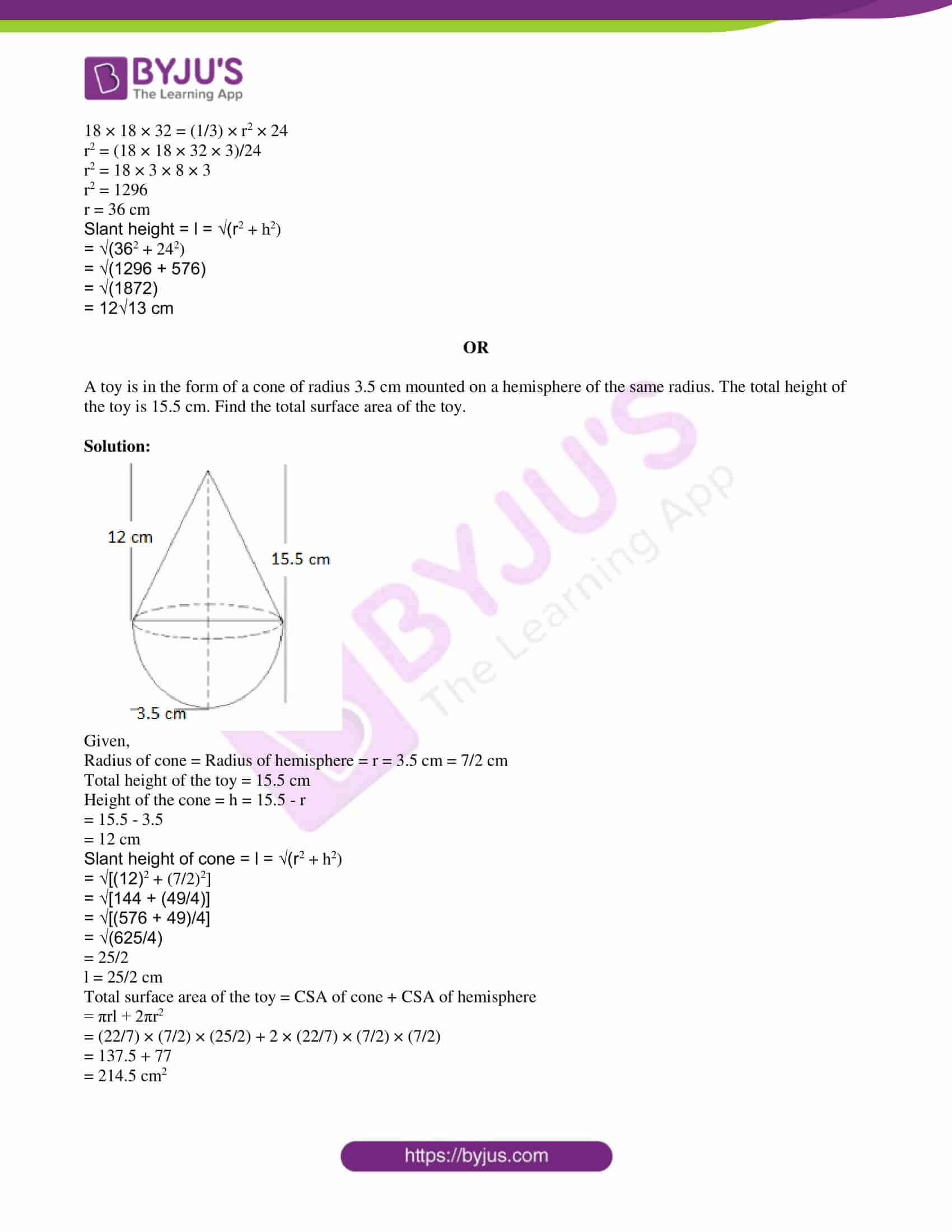Paper Code – 04/A

PART – A

1. (i) For which value of p does the pair of equations given below have a unique solution.

4x + py + 8 = 0

2x + 2y + 2 = 0

Solution:

Given,

4x + py + 8 = 0

2x + 2y + 2 = 0

Comparing with the standard form a1x + b1y + c1 = 0 and a2x + b2y + c2 = 0,

a1 = 4, b1 = p, c1 = 8

a2 = 2, b2 = 2, c2 = 2

Condition for a unique solution is:

a1/a2 ≠ b1/b2

4/2 ≠ p/2

2 ≠ p/2

p ≠ 4

Hence, p takes all the real values except 4.

(ii) For AP: 3/2, ½, -½, -3/2,…. Write the first term ‘a’ and common difference ‘d’.

Solution:

Given,

3/2, ½, -½, -3/2,….

First term = a = 3/2

Common difference = d = (½) – (3/2)

= (1 – 3)/2

= -2/2

= -1

Therefore, a = 3/2 and d = -1.

(iii) Write the definition of Pythagoras theorem.

Solution:

Pythagoras theorem states that: “In a right-angled triangle, the square of the hypotenuse side is equal to the sum of squares of the other two sides”.

(iv) Fill in the blank:

______ – cot2θ = 1

Solution:

cosec2θ – cot2θ = 1

(v) Find the volume of a sphere whose radius is 3 cm.

Solution:

Given,

Radius of sphere = r = 3 cm

Volume of sphere = (4/3)πr3

= (4/3) × (22/7) × 3 × 3 × 3

= (36 × 22)/7

= 792/7

= 113.14 cm3

PART – B

2. Find the LCM and HCF of 6 and 20 by prime factorisation method.

Solution:

Prime factorisation of 6:

6 = 2 × 3

Prime factorisation of 20:

20 = 2 × 2 × 5

LCM = 2 × 2 × 3 × 5 = 60

HCF = 2

3. Check whether the first polynomial is a factor of the second polynomial by dividing the second polynomial by first polynomial.

t2 – 3, t4 + 3t3 – 2t2 – 9t – 12

Solution: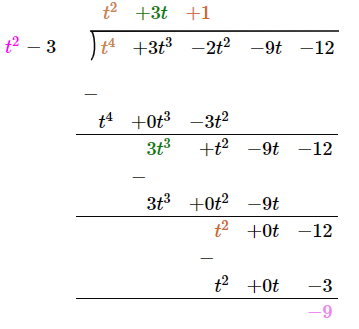Here, the remainder is not equal to 0.

Hence, the first polynomial is not a factor of the second polynomial.

4. Find such value of k for quadratic equation kx2 – 2kx + 6 = 0 so that they have two equal roots.

Solution:

Given,

kx2 – 2kx + 6 = 0

Comparing with the standard form ax2 + bx + c = 0,

a = k, b = -2k, c = 6

Condition of two equal roots of a quadratic equation is:

b2 – 4ac = 0

(-2k)2 – 4(k)(6) = 0

4k2 – 24k = 0

4k(k – 6) = 0

k = 0, k = 6

5. Show that a1, a2, a3,….an form an AP where an = 3 + 4n.

Solution:

Given,

an = 3 + 4n

Substituting n = 1,

a1 = 3 + 4(1) = 3 + 4 = 7

a2 = 3 + 4(2) = 3 + 8 = 11

a3 = 3 + 4(3) = 3 + 12 = 15

The series is: 7, 11, 15,….

This is an AP with a = 7 and d = 4.

OR

For AP: 7, 11, 15, 19,…. Find the sum of the first 15 terms.

Solution:

Given,

7, 11, 15, 19,….

First term = a = 7

Common difference = d = 11 – 7 = 4

n = 15

Sum of first n terms

Sn = n/2 [2a + (n – 1)d]

S15 = (15/2) × [2 × 7 + (15 – 1)4]

= (15/2) × [14 + 14 × 4]

= (15/2) × (14 + 56)

= (15/2) × 70

= 15 × 35

= 525

Hence, the sum of the first 15 terms of the given AP is 525.

6. The length of a tangent from a point A at distance 5 cm from the centre of the circle is 4 cm. Find the radius of the circle.

Solution:

Let PA be the tangent drawn from an external point P to the circle with centre O.

We know that the radius (OA) is perpendicular to the tangent (PA) through the point of contact (A).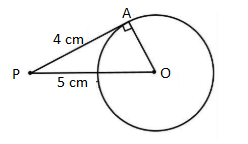In right triangle OAP,

OP2 = OA2 + PA2

(5)2 = OA2 + (4)2

OA2 = 25 – 16

= 9

OA = 3

Therefore, the radius of the circle is 3 cm.

OR

Two concentric circles are of radii 5 cm and 3 cm. Find the length of the chord of the larger circle which touches the smaller circle.

Solution:

Let O be the centre of two concentric circles.

AB be the chord of the larger circle which touches the smaller circle at P.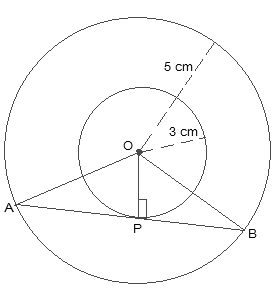OP = Radius of smaller circle = 3 cm

OA = OB = Radius of the larger circle = 5 cm

∴ OP ⊥ AB

In right triangle OPA,

By Pythagoras theorem,

OA2 = AP2 + OP2

52 = AP2 + 32

⇒ AP2 = 25 – 9 = 16

⇒ AP = 4

Also, the perpendicular from the center of the circle bisects the chord, i.e. AP will be equal to PB.

Thus, AB = 2AP = 2 × 4 = 8 cm

Hence, the length of the chord of the larger circle is 8 cm.

7. Draw a line segment of length 6.5 cm and divide it in the ratio 3 : 4.

Solution: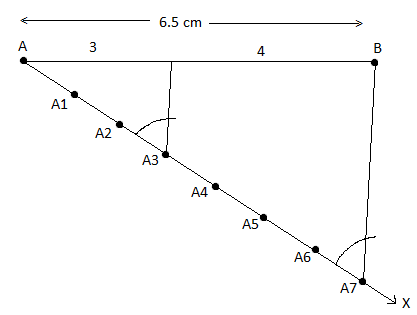OR

Draw a circle of radius 4 cm. From a point 7 cm away from a centre, construct the pair of tangents to the circle and measure its length.

Solution: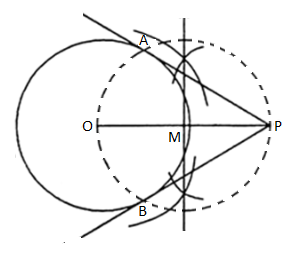Hence, PA and PB are the required tangents to the circle.

Length of the tangents = PA = PB = 5.75 cm (approx)

8. A bag contains 3 red and 5 black balls. A ball is drawn at random from the bag. What is the probability that the ball drawn is

(i) red

(ii) not red

Solution:

Given,

3 red balls ad 5 black balls

Total = 3 + 5 = 8

Total number of outcomes = 8

(i) Number of red balls = 3

P(getting a red ball) = ⅜

(ii) P(not getting a red ball) = 1 – P(getting a red ball)

= 1 – (⅜)

= (8 – 3)/8

= ⅝

PART – C

9. The cost of 1 pencil and 3 erasers is Rs. 10 and the cost of 4 pencils and 6 erasers is Rs. 28. Find the cost of 5 pencils and 4 erasers.

Solution:

Let x be the cost (in Rs) of one pencil and y be the cost (in Rs) of one eraser.

According to the given,

x + 3y = 10…..(i)

4x + 6y = 28….(ii)

From (i),

x = 10 – 3y….(iii)

Substituting (iii) in (ii),

4(10 – 3y) + 6y = 28

40 – 12y + 6y = 28

⇒ 6y = 40 – 28

⇒ 6y = 12

⇒ y = 2

Substituting y = 2 in (iii),

x = 10 – 3(2) = 10 – 6 = 4

Thus, the cost of one pencil is Rs. 4 and the cost of one eraser is Rs. 2.

The cost of 5 pencils and 4 erasers = 5(Rs. 4) + 4(Rs. 2)

= Rs. 20 + Rs. 8

= Rs. 28

10. Find the coordinates of the points of trisection (i.e. point dividing the three equal parts) of the segment joining the points A(2, -2) and B(-7, 4).

Solution:

Let the given points be:

A = (x1, y1) = (2, -2)

B = (x2, y2) = (-7, 4)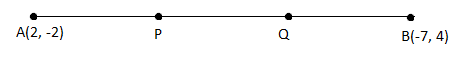P divides AB in the ratio 1 : 2.

Here, m : n = 1 : 2

Using the section formula,

P(x, y) = [(mx2 + nx1)/ (m + n), (my2 + ny1)/ (m + n)]

= [(-7 + 4)/ (1 + 2), (4 – 4)/ (1 + 2)]

= (-3/3, 0/3)

= (-1, 0)

Q is the midpoint of PB.

Q = [(-1 – 7)/2, (0 + 4)/2]

= (-8/2, 4/2)

= (-4, 2)

Hence, the required points are (-1, 0) and (-4, 2).

11. If tan (A + B) = √3 and tan (A – B) = 1/√3; 0° < A + B ≤ 90°; A > B, then find the value of A and B.

Solution:

Given,

tan (A + B) = √3

tan (A + B) = tan 60°

A + B = 60°….(i)

And

tan (A – B) = 1/√3

tan (A – B) = tan 30°

A – B = 30°….(ii)

2A = 90°

A = 90°/2 = 45°

Substituting A = 45° in (i),

45° + B = 60°

B = 60° – 45° = 15°

Therefore, A = 45° and B = 15°.

OR

Prove that √[(1 + sin A)/(1 – sin A)] = sec A + tan A; A < 90°.

Solution: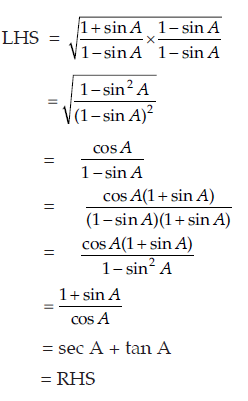12. Two poles of equal heights are standing opposite each other on either side of the road which is 80 m wide. From a point between them on the road the angles of elevation of top of the poles are 60° and 30°. Find the height of the poles and distance of the point from the poles.

Solution:

Let AB and CD be the two poles of equal heights.

BD = Distance between two poles.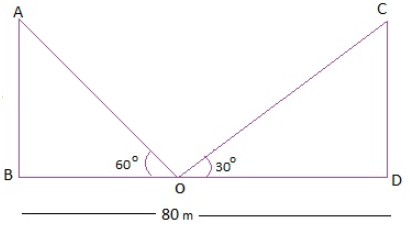OB + OD = 80 m

In right triangle CDO,

tan 30° = CD/OD

1/√3 = CD/OD

CD = OD/√3….(i)

In right ΔABO,

tan 60° = AB/OB

√3 = AB/(80 – OD)

AB = √3(80 – OD)

AB = CD (Given)

√3(80 – OD) = OD/√3 [From (i)]

3(80 – OD) = OD

240 – 3 OD = OD

4 OD = 240

OD = 60

Substituting OD = 60 in (i),

CD = OD/√3

CD = 60/√3

CD = 20√3 m

Also,

OB + OD = 80 m

⇒ OB = 80 – 60 = 20 m

Hence, the height of the poles is 20√3 m and distances from the point of elevation are 20 m and 60 m respectively.

13. In the figure given below a quadrilateral ABCD is drawn to circumscribe a circle. Prove that: AB + CD = AD + BC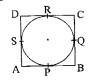Solution:

From the given,

DR = DS ….(i) (tangents from D)

BP = BQ ….(ii) (tangents from B)

AP = AS ….(iii) (tangents from A)

CR = CQ ….(iv) (tangents from C)

Adding (i), (ii), (iii) and (iv),

DR + BP + AP+ CR = DS + BQ + AS + CQ

By rearranging them,

(DR + CR) + (BP + AP) = (CQ + BQ) + (DS + AS)

AD + BC = CD + AB

Hence proved.

14. In a circle of radius 21 cm, an arc subtends an angle of 60° at the centre. Find

(i) the length of the arc

(ii) area of sector

Solution:

Given,

Angle of the sector = θ = 60°

(i) Length of an arc = θ/360° × 2πr

= (60°/360°) × 2 × (22/7) × 21

= (1/6) × 2 × (22/7) × 21

= 22 cm

(ii) Area of sector = (θ/360°) × πr2

= (60°/360°) × (22/7) × 21 × 21

= 11 × 21

= 231 cm2

OR

In the figure given below, find the area of the shaded region where a circular arc of radius 6 cm has been drawn with vertex O of an equilateral triangle OAB of side 12 cm as centre.Solution:

From the given,

OAB is an equilateral triangle since each angle is 60°.

Area of the sector is common in both.

Also,

Radius of the circle = r = 6 cm

Side of the triangle = 12 cm

Area of the equilateral triangle = √3/4 × (OA)2

= √3/4 × (12)2

= = √3/4 × 144

= 36√3 cm2

Area of the circle = πr2

= (22/7) × (6)2

= 792/7 cm2

Area of the sector making angle 60° = (60°/360°) × πr2

= (⅙) × (22/7) × (6)2

= 132/7 cm2

Area of the shaded region = Area of the equilateral triangle + Area of the circle – Area of the sector

= 36√3 + (792/7) – (132/7)

= 36√3 + (792 – 132)/7

= (36√3 + 660/7) cm2

15. The following distribution gives the daily income of 50 workers of a factory.

 Daily income (in Rs.) 100 – 120 120 – 140 140 – 160 160 – 180 180 – 200 No. of workers 12 14 8 6 10

Convert the above distribution to a less than type cumulative frequency distribution and draw its ogive.

Solution:

Less than type cumulative frequency distribution table:

 Daily income (in Rs.) Number of workers Less than 120 12 Less than 140 26 Less than 160 34 Less than 180 40 Less than 200 50

Plot the points (120, 12), (140, 26), (160, 34), (180, 40) and (200, 50).

Ogive: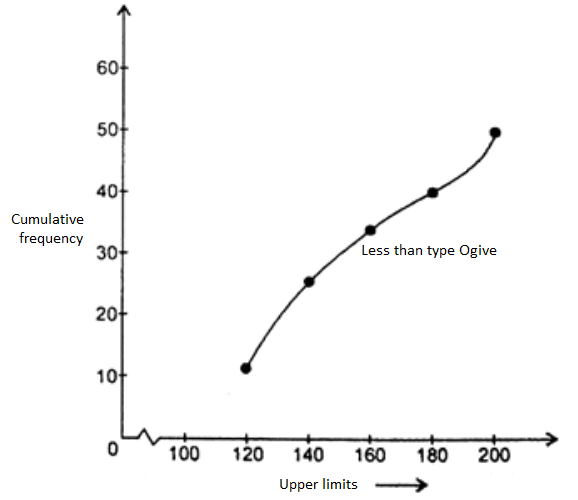OR

A survey conducted on 20 households in a locality by a group of students resulted in the following frequency table for the number of family members in a household.

 Family size 1 – 3 3 – 5 5 – 7 7 – 9 9 – 11 No.of families 7 8 2 2 1

Find the mode of this data.

Solution:

From the given,

Maximum frequency is 8 which lies in the class interval 3 – 5.

Modal class = 3 – 5

Lower limit of the modal class = l = 3

Frequency of the modal class = f1 = 8

Frequency of the class preceding the modal class = f0 = 7

Frequency of the class succeeding the modal class = f2 = 2

Class height = h = 2

Mode = l + [(f1 – f0)/ (2f1 – f0 – f2)] × h

= 3 + [(8 – 7)/ (2 × 8 – 7 – 2)] × 2

= 3 + [1/ (16 – 9)] × 2

= 3 + (1/7) × 2

= 3 + 0.286

= 3.286

Hence, the mode is 3.286.

PART – D

16. Prove that the ratio of the areas of two similar triangles is equal to the squares of the ratio of their corresponding sides.

Solution:

Given,

Two triangles ABC and PQR such that ∆ABC ~ ∆PQR

Draw altitudes AM and PN of two triangles ABC and PQR respectively.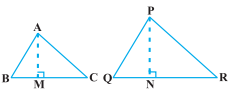ar(ΔABC) = 1/2 BC × AM

ar(ΔPQR) = 1/2 QR × PN

Now,

ar(ΔABC)/ar(ΔPQR) = [(1/2) BC × AM] / [(1/2) QR × PN]

= (BC × AM)/ (QR × PN) ….(i)

In ΔABM and ΔPQN,

∠B = ∠Q (∆ABC ~ ∆PQR)

∠M = ∠N (each 90°)

Thus, ∆ABM ~ ∆PQN (by AA similarity criterion)

⇒ AM/PN = AB/PQ ….(ii)

∆ABC ~ ∆PQR (given)

AB/PQ = BC/QR = CA/RP ….(iii)

From (i) and (iii),

ar(ΔABC)/ar(ΔPQR) = (AB/PQ) × (AM/PN)

= (AB/PQ) × (AB/PQ) [From (ii)]

= (AB/PQ)2

Similarly,

ar(ΔABC)/ar(ΔPQR) = (AB/PQ)2 = (BC/QR)2 = (CA/RP)2

Hence proved.

OR

Prove that in a right triangle, the square of the hypotenuse is equal to the sum of the squares of the other two sides.

Solution:

Given:

In a right triangle ABC, ∠B = 90°

To prove:

AC2 = AB2 + BC2

Construction:

Draw a perpendicular BD onto the side AC.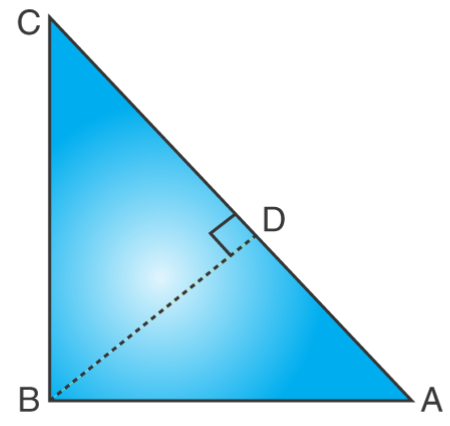We know that,

Therefore, AD/AB = AB/AC (by similarity)

AB2 = AD × AC …..(i)

Also, △BDC ~△ABC

Therefore, CD/BC = BC/AC (by similarity)

BC2 = CD × AC ….(ii)

AB2 + BC2 = AD × AC + CD × AC

AB2 + BC2 = AC (AD + CD)

Since, AD + CD = AC

Therefore, AC2 = AB2 + BC2

Hence proved.

17. A cylindrical bucket 32 cm high and with a radius of base, 18 cm is filled with sand. This bucket is emptied on the ground and a conical heap of sand is formed. If the height of the conical heap is 24 cm, find the radius and slant height of the heap.

Solution:

Given,

Height of the cylindrical bucket = H = 32 cm

Radius of the base of cylindrical bucket = R = 18 cm

Height of the conical heap = h = 24 cm

Let r be the radius of the base of conical heap.

Volume of sand in cylindrical bucket = Volume of sand of conical heap

πR2H = (1/3)πr2h

18 × 18 × 32 = (1/3) × r2 × 24

r2 = (18 × 18 × 32 × 3)/24

r2 = 18 × 3 × 8 × 3

r2 = 1296

r = 36 cm

Slant height = l = √(r2 + h2)

= √(362 + 242)

= √(1296 + 576)

= √(1872)

= 12√13 cm

OR

A toy is in the form of a cone of radius 3.5 cm mounted on a hemisphere of the same radius. The total height of the toy is 15.5 cm. Find the total surface area of the toy.

Solution: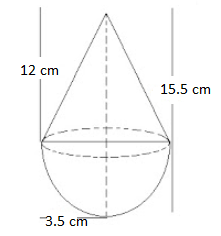Given,

Radius of cone = Radius of hemisphere = r = 3.5 cm = 7/2 cm

Total height of the toy = 15.5 cm

Height of the cone = h = 15.5 – r

= 15.5 – 3.5

= 12 cm

Slant height of cone = l = √(r2 + h2)

= √[(12)2 + (7/2)2]

= √[144 + (49/4)]

= √[(576 + 49)/4]

= √(625/4)

= 25/2

l = 25/2 cm

Total surface area of the toy = CSA of cone + CSA of hemisphere

= πrl + 2πr2

= (22/7) × (7/2) × (25/2) + 2 × (22/7) × (7/2) × (7/2)

= 137.5 + 77

= 214.5 cm2